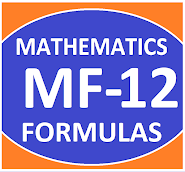## Posts

Showing posts from November, 2019

### Common Errors in Secondary Mathematics

Common Errors Committed  by the  Students  in Secondary Mathematics   Errors  that students often make in doing secondary mathematics  during their practice and during the examinations  and their remedial measures are well explained here stp by step.  Some Common Errors in Mathematics

### Triangle Properties And Types GeometryTRIANGLES ITS PROPERTIES AND TYPES Triangle:- A polygon with three sides is called a triangle. Triangle is a closed figure which have three sides, three angles and three vertices. Classification of triangles on the bases of sides These are of three types:- Scalene Triangle Isosceles Triangle Equilateral triangle Scalene Triangle:-   A triangle whose all sides are different is called a scalene triangle. Isosceles Triangle:- A triangle whose two sides are equal is called isosceles triangle. Equilateral Triangle:- A triangle whose all sides are equal is called an equilateral triangle. Classification of triangles on the basis of angles. These are of three types:- Acute angled Triangle Right angled Triangle Obtuse angled Triangle   Acute angled triangle:- A triangle whose all angles are acute is called acute angled triangle. Right angled Triangle:- A triangle whose one angle equal

### Class XII Maths Formulas | Basics | AssignmentsChapter-wise Basics and Formulas CBSE syllabus, sample papers, unit tests and solutions of sample papers, CBSE syllabus for class XII for all subjects, chapter-wise basic concepts of mathematics for class XII, chapter-wise planning in mathematics for class XII. Mathematics MCQ Class XII  Click Here Maths Practical for Classes   XI & XII Click Here   Maths Project Work For Class XII Click Here ******************************************* TEACHERS NOTES SUBJECT: MATHEMATICS CLASS XII CLASS 12 (Non- Medical and Applied Mathematics) Chapter 1 Chapter 2 Chapter 3 Chapter 4 Chapter 5 Chapter 6 Chapter 7 Chapter 8 Chapter 9 Chapter 10 Chapter 11 Chapter 12 Chapter 13 Applied   Maths *************************************** RESOURCE CENTRE MATHEMATICS CLASS XII

### Lesson Plan Math Class X (Ch-10) | Tangent To a CircleE- LESSON PLAN    SUBJECT MATHEMATICS     CLASS 10 lesson plan for maths class X chapter 10 circle, lesson plans for mathematics teachers,  Method to write lesson plan for maths class 10, lesson plan for maths class X, lesson plan for mathematics grade X, lesson plan for maths teacher in B.Ed. TEACHER'S NAME  :   Dinesh Kumar SCHOOL :  RMB DAV CENTENARY PUBLIC SCHOOL NAWANSHAHR SUBJECT   :    MATHEMATICS CLASS                  :    X  STANDARD BOARD                 :    CBSE LESSON TOPIC / TITLE   :  CHAPTER 10:  TANGENTS TO A CIRCLE ESTIMATED DURATION:  This chapter is divided into Eight modules and it is completed in Ten class meetings. TOPIC    Chapter 10 : Circle

### Lesson Plan Math Class 12 Ch-13 | ProbabilityE- LESSON PLAN    SUBJECT MATHEMATICS     CLASS 10+2 Lesson Plan, Class XII Subject Mathematics, chapter 13, Probability , for Mathematics Teacher. Effective way of Teaching Mathematics. Top planning by the teacher for effective teaching in the class. E lesson planning for mathematics. Board – CBSE CLASS –XII SUBJECT- MATHEMATICS CHAPTER 13  :- Probability TOPIC:- CHAPTER 13 : Probability DURATION:-    This chapter is divided into 7 modules and is completed in 30 class meetings. PRE- REQUISITE KNOWLEDGE:- Knowledge of probability class IX & X Knowledge of probability class XI TEACHING AIDS:-   Green Board, Chalk,   Duster, Charts, smart board, projector, laptop etc. METHODOLOGY:-    Lecture method OBJECTIVES:- Conditional probability. Multiplication Theorem on probability. Independent events, mutually exclusive events and exhaustive events. Total probability , and Bayes th